# 基于可见光遥感数据的海洋内波垂向重构Internal Wave Vertical Rebuilding Based on CCD Remote Sensing Data

• 全文下载: PDF(1460KB)    PP.282-290   DOI: 10.12677/CSA.2019.92033
• 下载量: 150  浏览量: 242   国家科技经费支持

Since traditional internal wave parameters inversion based on remote sensing images is usually limited to the texture information of ocean surface, or the amplitude of internal wave is derived from the internal wave profile, it is difficult to describe the three-dimensional structure of internal wave, which limits the underwater application of ocean internal wave. In this paper, a new method for reconstruction of internal waves’ two-dimensional section is given by using internal wave-length and the horizontal information of internal wave, receiving internal wave-amplitude and vertical mode functions combined with the background density information. After taking full consideration to the relationship between the internal waves’ surface flow field which is reflected by the visible image and the internal wave fluctuation, the maximum vertical amplitude (at the thermocline) and the vertical mode function of internal waves, the two-dimensional vertical section of internal waves can be reconstructed in real time, which provides an accurate underwater distribution of internal waves for underwater environmental applications.

1. 引言

2. 数据准备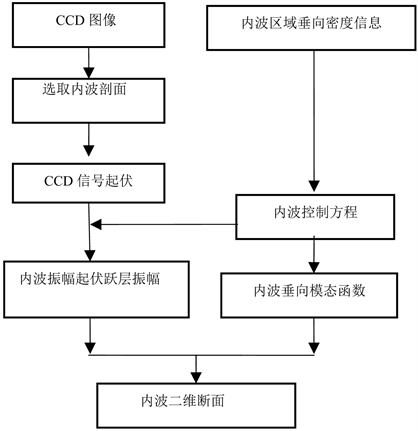Figure 1. Data processes flow

2.1. 内波剖面数据获取

CCD可见光遥感图像是内波特征参数振幅、波长等反演的基础，反射率可以反应海面散射特性，进而反应海面流场辐聚辐散规律，由于内波到达海面时振幅基本为0  ，因此可通过信号剖面得到内波表面流场的分布规律，进而得到内波振幅在断面中的起伏规律。本文所用可见光数据是由中国资源卫星应用中心提供的HJ数据，获取时间为2010年7月2日，数据地理位置为 $\text{E}108˚00\text{'}58\text{'}\text{'}~\text{E}112˚48\text{'}28\text{'}\text{'}$$\text{N}19˚09\text{'}56\text{'}\text{'}~\text{N}22˚59\text{'}15\text{'}\text{'}$ ，经向分辨率为30.00 m，纬向分辨率为30.00 m，如图2所示。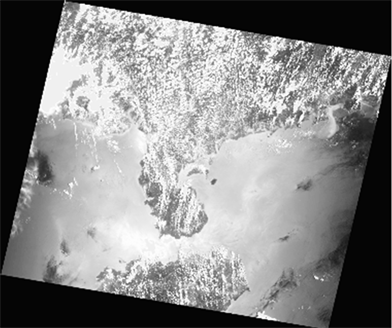Figure 2. CCD data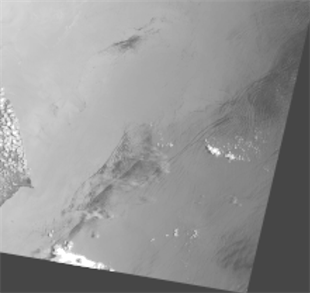Figure 3. CCD data to select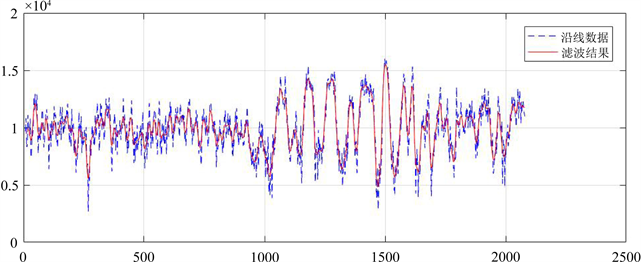Figure 4. CCD data signal fluctuation

2.2. 内波振幅

2.3. 内波垂向模态函数

${W}^{″}-\frac{{N}^{2}}{g}{W}^{\prime }+\frac{{N}^{2}-{\omega }^{2}}{{\omega }^{2}-{f}^{2}}{k}_{h}^{2}W=0$ (1)

$\left\{\begin{array}{l}\frac{{\partial }^{2}W}{\partial {z}^{2}}-{k}^{2}\left(1-\frac{{N}^{2}}{{\omega }^{2}}\right)W=0\\ W\left(0\right)=W\left(-H\right)=0\end{array}$ (2)

k为水平波数。令：${N}^{2}\left(z\right)={b}^{2}\left(z\right)$ ，则方程变为：

$\left\{\begin{array}{l}\frac{{\text{d}}^{2}W}{\text{d}{z}^{2}}+\left\{\lambda {b}^{2}\left(z\right)-{k}^{2}\right\}W=0\\ W\left(0\right)=W\left(-H\right)=0\end{array}$ (3)(4)

3. 内波二维模型的构建

3.1. 垂向标准层振幅的构造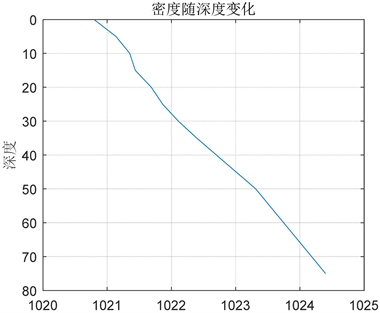Figure 5. The density section in experiment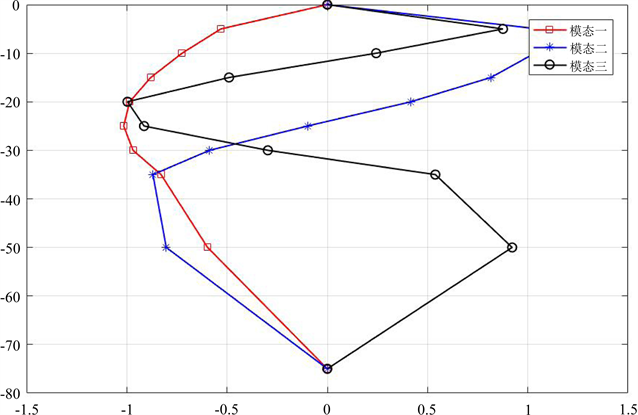Figure 6. Vertical mode functions in experiment

3.2. 垂向标准层无量纲波动起伏反演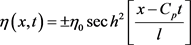(5)

$E=A\mathrm{sec}{h}^{2}\left[\frac{x-{C}_{p}t}{l}\right]\mathrm{tanh}\left[\frac{x-{C}_{p}t}{l}\right]$ (6)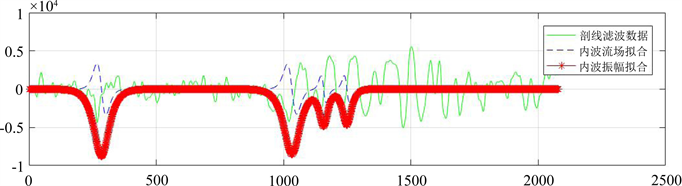Figure 7. Distribution of dimensionless internal waves along the section

3.3. 内波二维断面重构

$\eta \left(lon,lat,z\right)$ 为随位置变化的内波引起的海水位移，则有：

$\eta \left(lon,lat,z\right)=\left[C1\left(1,z\right)\cdot A\right]\cdot \theta \cdot C2\left(1,z\right)=A\cdot \theta \cdot C1\cdot C2$ (7)

4. 结论

1) 在内波垂向模态函数的分解中，根据内波理论和以往测量经验获知第一模态是主导模态，但不可否认还有第二模态、第三模态等后续模态的存在。由于无法解得各个垂向模态函数在实际内波中所占的权重比例，本文只用第一模态函数重构内波二维断面，重构模型尚不够精细。若能用尽量多的垂向模态函数权重叠加来代表内波区的垂向分布结构，则重构结果将更接近实际。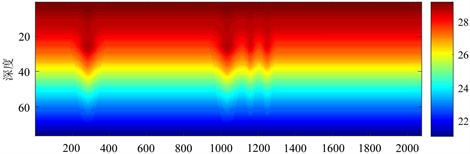(a) 温度重构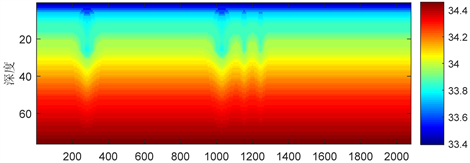(b) 盐度重构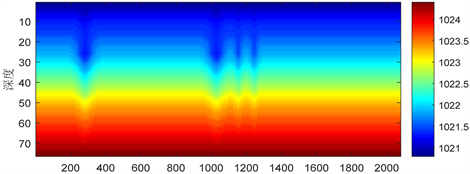(c) 密度重构

Figure 8. Vertical reconstruction of internal wave

2) 在内波垂向密度剖面提供方面，本文采用空间分辨率为 $1{5}^{\prime }×1{5}^{\prime }$ 的月平均背景场获取内波发生处海水剖面数据，在时间和空间上都不够精确，若能同时获得当时当地的可见光数据，重构结果将更加符合实际。

  Bai, X., Liu, Z., Li, X., et al. (2014) Generation Sites of Internal Solitary Waves in the Southern Taiwan Strait Revealed by MODIS True-Colour Image Observations. International Journal of Remote Sensing, 35, 4086-4098. https://doi.org/10.1080/01431161.2014.916453  da Silva, J.C.B., Buijsman, M.C. and Magalhaes, J.M. (2015) Internal Waves on the Upstream Side of a Large Sill of the Mascarene Ridge: A Comprehensive View of Their Generation Mechanisms and Evolution. Deep Sea Research Part I: Oceanographic Research Papers, 99, 87-104. https://doi.org/10.1016/j.dsr.2015.01.002  Ostrovesky, L.A. and Stepanyants, Y.A. (1989) Do Internal Solitions Exist in the Ocean. Reviews of Geophysics, 7, 293-310. https://doi.org/10.1029/RG027i003p00293  Bai. X., Liu. Z/, Li. X/, et al. (2014) Generation Sites of Internal Solitary Waves in the Southern Taiwan Strait Revealed by MODIS True-Colour Image Observations. In-ternational Journal of Remote Sensing, 35, 4086-4098. https://doi.org/10.1080/01431161.2014.916453  da Silva, J.C.B., Buijsman, M.C. and Magalhaes, J.M. (2015) Internal Waves on the Upstream Side of a Large Sill of the Mascarene Ridge: A Comprehensive View of Their Generation Mechanisms and Evolution. Deep Sea Research Part I: Oceanographic Research Papers, 99, 87-104. https://doi.org/10.1016/j.dsr.2015.01.002  史璐, 王晶, 梅源. 基于MODIS遥感影像的直布罗陀海峡内波传播特性研究[J]. 激光与电子学进展, 2018, 55(1): 386-392.  王晶, 郭凯, 孙美玲, 等. 3层模型的内波传播方程与参数反演[J]. 遥感学报, 2015, 19(2): 188-194.  陈维亮, 陈标, 王丹, 等. 基于实测资料的内波振幅修正参数[J]. 中国海洋大学学报自然科学版, 43(1): 11-16.  孙丽娜, 张杰, 孟俊敏, 等. 基于多源遥感数据的日本海内波特征研究[J]. 海洋学报, 2018, 40(3): 102-111.  邓冰, 张翔, 张铭. 背景流中海洋内波垂向结构[J]. 海洋科学进展, 2014, 32(2): 121-129.  Zha, G.Z., He, Q.Y., Guan, C.L., et al. (2016) Speckle Suppression in Synthetic Aperture Radar Ocean Internal Solitary Wave Images with Curvelet Transform. Acta Oceanologica Sinica, 35, 13-21. https://doi.org/10.1007/s13131-016-0929-3  Wang, J., Sun, M.L., Zhang, X.D., et al. (2015) A Numerical Study of the Gen-eration Mechanism of Internal Solitary Waves in the Luzon Strait. Acta Oceanologica Sinica, 34, 38-43. https://doi.org/10.1007/s13131-015-0695-7